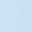Discussion about math, puzzles, games and fun.   Useful symbols: ÷ × ½ √ ∞ ≠ ≤ ≥ ≈ ⇒ ± ∈ Δ θ ∴ ∑ ∫  π  -¹ ² ³ °

You are not logged in.

## #1 2020-03-23 22:14:26

666 bro
MemberFrom: Flatland
Registered: 2019-04-26
Posts: 586

### Logarithms

How would we calculate logarithms of base -10 with any number  without using log tables and by hand?

"An equation for me has no meaning, unless it expresses a thought of God"- Srinivasa ramanujan

Offline

## #2 2020-03-24 02:11:30

BobRegistered: 2010-06-20
Posts: 8,914

### Re: Logarithms

hi 666 bro,

In general you couldn't do this by hand because the calculations would be too complicated.(power series for log)  But some values can be determined.

eg.  log 10 = 1
log 100 = 2
log 1000 = 3
log(0.1) = -1

log √10 = 0.5 because to multiply two numbers you add their logs.

When I was at school (before modern calculators!) we had to learn that log 2 ≈ 0.3010,  log 3 ≈ 0.4771 and log (pi) ≈ 0.4972

So I could get log 4, log 6, log 9, log 2pi etc.

As a class exercise when I became a teacher, prior to 'doing' logs for the first time, we would make our own slide rules by making two strips and marking 1 at one end and 1000 at the other.  You can mark on 10 and 100 at equal intervals between, so, in effect, the ruler is 'measuring' logs.  Then estimate the position for 32 by saying 1024 ≈ 1000 so 32 is half way along.  And 2 is 1/5 of the way between 1 and 32 (2^5 = 32) so then you can use that to estimate 5 (log 5 = log 10 - log 2).  By marking the same log scale on both strips you can transfer measurements from one to the other and hence get 64, 50, 500, 640 and so on. Estimate the position for 49 as it's nearly 50, and hence 7.  81 is close to 80, so then 9 and then 3.  All the time practising the rules of logs by discovery.

Bob

Children are not defined by school ...........The Fonz
You cannot teach a man anything;  you can only help him find it within himself..........Galileo Galilei
Sometimes I deliberately make mistakes, just to test you!  …………….BobOffline